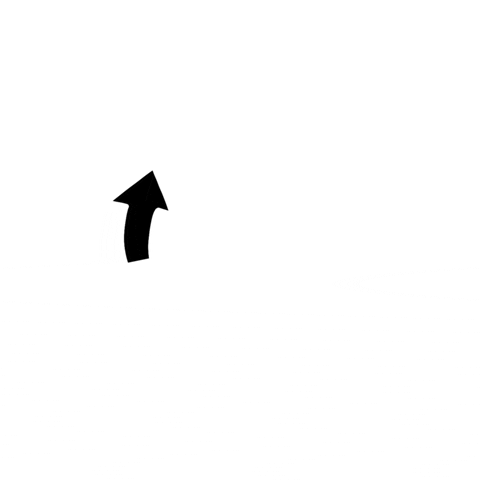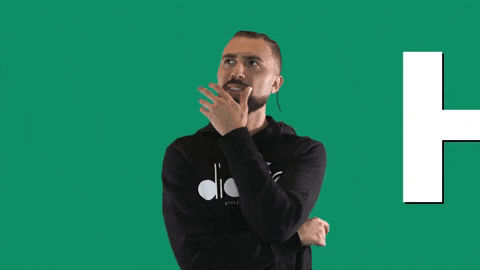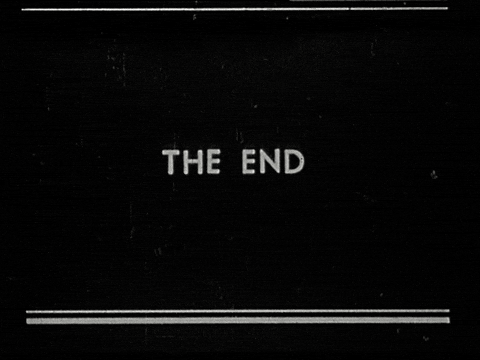# CyclesWhen an action needs to be repeated a large number of times, cycles are used in programming. For example, you need to display the text 📜 "Hello, World!" 🌎. Instead of repeating the same text output command 📜 two hundred times, a loop is often created that repeats 200 times what is written 🖊️ in the body of the loop. Each repetition is called an iteration.Iteration in programming - in the broadest sense - the organization of data processing in which actions are repeated many times, without leading to calls 📞 themselves (as opposed to recursion). In a narrow sense, it is one step in an iterative, cyclical process.

A loop in JavaScript (and in all other languages👅) is a block of code📟 that repeats itself as long as a known condition is true. There are many different types of loops, but they all essentially do the same thing: they repeat an action several times.

## The while() loop​The `while` operator creates a loop that executes the specified instruction while the condition being tested is true. The logical value of the condition is calculated вычис before executing the loop body.

### Syntax​``while (condition) {  instruction // algorithm code - instructions}``

`condition` is an expression whose boolean value is checked each time before entering the loop. If the value is true - `true`✅, then the instruction is executed. When the value becomes false - `false`❎, we exit the loop.

An instruction is an algorithm code that is executed every time the condition is true. To execute multiple instructions in a loop, use the `{...}` block operator to group them. When using 1 command in the body of the loop, curly braces can be omitted.

Here is a simple example of a loop `while (condition) {...}`

Live Editor
Result

The `count` in the example is `0`. The condition of our loop (it is written скоб in brackets) is that the block with the code will repeat itself over and over until (that is, the actual `while`) `count` is less than `10`.

Since the initial value of `0` is less than` 10`, the code📟 runs. Each time the interpreter re-checks the ✔️ condition, if the condition is still true, then the code📟 will be run again. Each time we increment the counter value by `1`. Otherwise, the value would remain at `0`, so our condition `counter <10` would always remain true, and our code📟 would loop forever!

As a result, after `10` cycles it will become `10`. After that, the interpreter will terminate the loop since the condition is `false` ❎ and go to the final lines of our code📟.

## The for() loop​The `for` expression creates a loop of three 3 optional expressions in parentheses, separated by semicolons.

### Syntax​``for ([initialization]; [condition]; [final expression])   { expression }``

`initialization` - expression or definition of variables. This expression can optionally declare new variables using the `let` keyword. These variables are visible only in the `for` loop, i.e. in the same scope (for security).

`condition` is an expression that is executed at each iteration of the loop. If the expression is true, the loop is executed. The condition is optional. If not, the condition is always considered true. If the expression is false, execution of `for` is terminated.

`final expression` - an expression that is executed at the end of the loop iteration. Occurs until the next condition is met. Usually used to `increment ++`, `decrement -` or `update i + = 5` of a counter variable 🔔.

`expression` - executable code of the algorithm while the condition of the loop is `true`. To execute multiple expressions in a loop, use the `{...}` block to group those expressions. To avoid executing any expression in a loop, use an empty `for (;;;)` expression.

Let's calculate the sum of numbers from 0 to 100 👇:

Live Editor
Result

Remember when we wrote our 1️⃣ first `while()` what happened to our counter? We found that it is very important that it constantly changes (`increment ++` helped us with this). Because if you forget about it, then the code📟 will fall into an `infinite loop of loops`.

Well, situations like this happen quite regularly with `while-loops`, which is why `for` was done with a built-in counter!

### Example for loop​When you first see the syntax📖 of a `loop for`, you might think 🤔 that this is something very strange. But you should still study it 🧑‍🎓, since you will meet like this many times:

Live Editor
ResultWell, did you know? They should have! After all, this is practically the same code📟 that we used for the `while` loop at the beginning of the chapter! The three parts of the cycle are separated by semicolons; they were all in that while loop, but in different places. Let's take a closer look:1. First, we declare a counter variable - `let i = 0`. It is in the function itself and outside this loop that this `i` will be absent, and this is safe!
2. Next, we set a condition that the interpreter will check before each iteration of the loop (to determine whether it is worth starting the code at all). An iteration is called one iteration of the loop (for example, if we had 10 startup cycles, then we can say that there were 10 code iterations).
3. The third part of our code is `increment ++` (or `decrement --`). It runs at the end of each iteration to change our variable every time 🔔.

## Conclusion​Almost always, when you know the number of iterations needed, you would rather work with for than with while. This is why `for` loops are so popular. There are other cycles, but they are not so popular and if you want you can get acquainted with them here.

## Problems?​Write to Discord chat.

## Questions:​What is the name of a block of code that repeats itself over and over again until a given condition is no longer true?

1. Cycle
2. Condition
3. Initialization

Which of the two loop operators is more popular?

1. `for`
2. `while`
3. `break`

How many messages will the following code output to the console?

``let k = 0while (k < 7) {  console.log('one more line!')}``
1. `7`
2. `8`
3. `infinity`

What is the character used to separate the parts of the for loop that are in parentheses?

1. `&&`
2. `;`
3. `=!`

If `_______` always remains true, then the code can get stuck in an infinite `_______`.

1. condition / loop
2. cycle / condition

What is the middle `_____` of the three bracketed parts of a `for` loop called?

1. Expression
2. Condition
3. Increment

How many messages will the following code output to the console?

``const maxNumer = 17let n = 0while (n <= maxNumer) {  console.log("Let's count!" + n)  n = n + 2}``
1. `8`
2. `9`
3. `10`

What is the term for a single (step) passage of the cycle?

1. Iteration
2. Interrupt
3. Looping

In order to understand how much you learned this lesson, take the test in the mobile application of our school on this topic or in our telegram bot.## Contributors ✨​

Thanks goes to these wonderful people (emoji key):Dmitriy K.📖Dmitriy Vasilev💵Resoner2005🐛 🎨 🖋Navernoss🖋 🐛 🎨